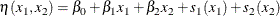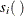### Forms of Additive Models

Suppose that y is a continuous variable and x1 and x2 are two explanatory variables of interest. To fit an additive model, you can use a MODEL statement similar to that used in many regression procedures in the SAS System:

model y = spline(x1) spline(x2);


This model statement requires the procedure to fit the following model:where theterms denote nonparametric spline functions of the respective explanatory variables.

The GAM procedure can fit semiparametric models. The following MODEL statement assumes a linear relation with x1 and an unknown functional relation with x2:

model y = param(x1) spline(x2);


If you want to fit a model containing a functional two-way interaction between x1 and x2, you can use the following MODEL statement:

model y = spline2(x1,x2);


In this case, the GAM procedure fits a model equivalent to that of PROC TPSPLINE.